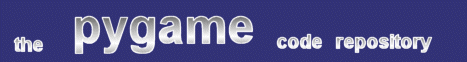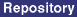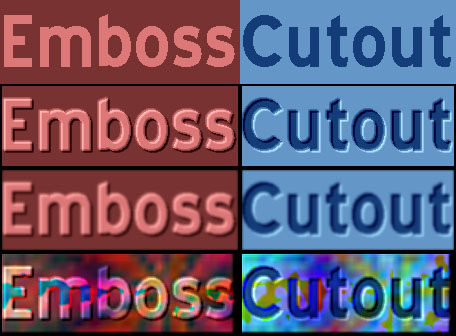### Title: Emboss / Cutout Text Effects

Author: Sherwyn Ramkissoon (bei at sympatico.ca)
Submission date: December 18, 2001

Description: A script that demonstrates several common image manipulation effects on a text string.pygame version required: Any (with numeric)
SDL version required: Any
Python version required: Any

Comments: This is a pretty terrifyingly complex script that uses Numeric to generate some very attractive filter effects on some text. It's not actually a module, so you can't really import and reuse it, but the necessary fixes to allow that are pretty simple. The overloading of the word 'filter' as a function name and argument doesn't necessarily conflict with the built-in python keyword 'filter', but reduces readability somewhat. Still, very pretty.

```
# cutout.py
#
# Having recently discovered Python, this looked like a
# fun way to learn the language...
#
# Uses 5x5 convolution matrices to do Emboss, Cutout, Blur & Edge effects.
# Generates a somewhat random circular gradient texture.
#
# Even using Numpy this is pretty slow.
#
# Bits of code blatantly stolen from the Pygame site.
#
# Author: Sherwyn Ramkissoon (bei@sympatico.ca)

# Import some useful (or not) stuff
import os
import sys
import pygame
import pygame.image
import pygame.transform
import pygame.font
import Numeric as N
import math as math
import time as time
import random as random
import pygame.surfarray as surfarray
from pygame.locals import *

# FILTER STUFF

# Convolution Kernels
blurFilter = [[0,1,2,1,0],[1,2,4,2,1],[2,4,8,4,2],[1,2,4,2,1],[0,1,2,1,0]]
blurDiv = 48
cutoutFilter = [[-4,-3,-2,0,0],[-3,-2,-1,0,0],[-2,-1,18,1,2],[0,0,1,2,3],[0,0,2,3,4]]
embossFilter = [[4,3,2,0,0],[3,2,1,0,0],[2,1,18,-1,-2],[0,0,-1,-2,-3],[0,0,-2,-3,-4]]
cutDiv = 18
edgeFilter = [[0,0,0,0,0],[0,0,-1,0,0],[0,-1,5,-1,0],[0,0,-1,0,0],[0,0,0,0,0]]
edgeDiv = 1

# 5x5 Convolution filter
def Filter(inarray,filter,divisor):
size = len(filter)
out = N.zeros(inarray.shape)
(R,C,D) = out.shape
for i in range((1-size)/2,(size+1)/2):
for j in range((1-size)/2,(size+1)/2):
if filter[i+2][j+2] != 0:
out[2:R-2,2:C-2] += inarray[2+i:R-2+i,2+j:C-2+j]*filter[i+2][j+2]
out = N.clip(out/divisor,0,255)
return out

# Blur Filter
def Blur(inarray):
return Filter(inarray,blurFilter,blurDiv)

# If font color is lighter than background, Cutout produces an embossed effect.
# If font color is darker than background, Cutout produces a cutout effect.
def Cutout(inarray):
return Filter(inarray,cutoutFilter,cutDiv)

# The opposite of Cutout
def Emboss(inarray):
return Filter(inarray,embossFilter,cutDiv)

def Edge(inarray):
return Filter(inarray,edgeFilter,edgeDiv)

# Apply Filter to surface
def Effect(surf,filterFunc):
pix = pygame.surfarray.array3d(surf)
surf = pygame.Surface((pix.shape, pix.shape), 32)
surfarray.blit_array(surf,filterFunc(pix))
return surf

def R(m):
return random.uniform(0,m)

# Silly gradient function using polar coordinates
g = int(math.fabs((255-noise)*math.cos(rad/50.0+phase)) + R(noise))
r = int(math.fabs((255-noise)*math.cos(freq*theta+phase)) + R(noise))
b = int(math.fabs((255-noise)*math.sin(freq*theta/2+phase)) + R(noise))
return (r,g,b)

# Gradient Generator - Generates some pixels & interpolates the rest
# Screen seems to be in column-major order
bg = N.zeros((W,H,3))
for i in range(0,W,step):
xpos = i-W/2-Cx
for j in range(0,H,step):
ypos = j-H/2-Cy
theta = math.atan2(ypos,xpos)
# Interpolate along y
ns = step
while (ns > 1):
ns2 = ns/2
bg[0::step,ns2:-ns2:ns,:] = (bg[0::step,0:-ns:ns,:] + bg[0::step,ns::ns,:])/2
ns = ns2
# Interpolate along x
ns = step
while (ns > 1):
ns2 = ns/2
bg[ns2:-ns2:ns,:,:] = (bg[0:-ns:ns,:,:] + bg[ns::ns,:,:])/2
ns = ns2
return bg

# Couldn't get alpha to work, so simulate it (slowly) :-|
def CalcAlpha(inarray):
return inarray

# Apply pseudo-alpha gradient
def PAlpha(surf):
pix = pygame.surfarray.array3d(surf)
pix = N.array(pix,N.Int16)
pAlpha = N.ones(pix.shape)*255
pAlpha = CalcAlpha(pAlpha)
pix = (pAlpha*pix)/255
try:
pix = (pix*255)/N.argmax(N.argmax(N.argmax(pix)))
except:
pass
surf = pygame.Surface((pix.shape, pix.shape), 32)
surfarray.blit_array(surf,pix)
return surf

# MAIN PROGRAM

# Setup	Pygame
pygame.init()
font = pygame.font.Font(None, 90)
#font = pygame.font.Font("c:/windows/fonts/stencil.ttf",80)

# Generate Text
text = []
print "Thinking..",
text.append(font.render("Emboss",0,(220,120,120),(120,50,50)))
print "\b.",
text.append(font.render("Cutout",0,(20,60,120),(100,150,200)))
print "\b.",
text.append(Effect(text,Cutout))
print "\b.",
text.append(Effect(text,Cutout))
print "\b.",
text.append(Effect(text,Blur))
print "\b.",
text.append(Effect(text,Blur))
print "\b.",
text.append(Effect(Effect(Effect(PAlpha(text),Cutout),Blur),Edge))
print "\b.",
text.append(Effect(Effect(Effect(PAlpha(text),Cutout),Blur),Edge))
print "Done!",

# Setup screen
H = text.get_height()*len(text)/2
W = text.get_width() + text.get_width()
screen = pygame.display.set_mode((W,H), 0, 32)
pygame.display.set_caption("Filter Effects")

# Display everything
for i in range(len(text)):
screen.blit(text[i], ((i%2)*text.get_width(),(int(i/2))*text.get_height()) )
pygame.display.flip()

#wait for the finish
while 1:
event = pygame.event.wait()
if event.type is KEYDOWN and event.key == K_s: #save it
name = os.path.splitext(sys.argv) + '.bmp'
print 'Saving image to:', name
pygame.image.save(screen, name)
elif event.type in (QUIT,KEYDOWN,MOUSEBUTTONDOWN):
break

```

 Feedback